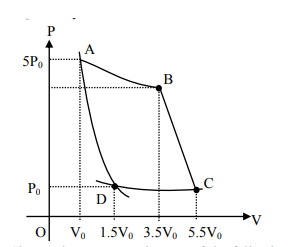Deepak Scored 45->99%ile with Bounce Back Crack Course. You can do it too!

# In the reported figure, there is a cyclic process ABCDA on a sample of 1 mol of a diatomic gas.

Question:

In the reported figure, there is a cyclic process ABCDA on a sample of $1 \mathrm{~mol}$ of a diatomic gas. The temperature of the gas during the process $\mathrm{A} \rightarrow \mathrm{B}$ and $\mathrm{C} \rightarrow \mathrm{D}$ are $\mathrm{T}_{1}$ and $\mathrm{T}_{2}\left(\mathrm{~T}_{1}>\mathrm{T}_{2}\right)$ respectively.Choose the correct option out of the following for work done if processes BC and DA are adiabatic.

1. $\mathrm{W}_{\mathrm{AB}}=\mathrm{W}_{\mathrm{DC}}$

2. $W_{A D}=W_{B C}$

3. $\mathrm{W}_{\mathrm{BC}}+\mathrm{W}_{\mathrm{DA}}>0$

4. $\mathrm{W}_{\mathrm{AB}}<\mathrm{W}_{\mathrm{CD}}$

Correct Option: , 2

Solution:

Work done in adiabatic process $=\frac{-n R}{\gamma-1}\left(T_{f}-T_{i}\right)$

$\therefore \mathrm{W}_{\mathrm{AD}}=\frac{-\mathrm{nR}}{\gamma-1}\left(\mathrm{~T}_{2}-\mathrm{T}_{1}\right)$

and $\mathrm{W}_{\mathrm{BC}}=\frac{-\mathrm{nR}}{\gamma-1}\left(\mathrm{~T}_{2}-\mathrm{T}_{1}\right)$

$\therefore \mathrm{W}_{\mathrm{AD}}=\mathrm{W}_{\mathrm{BC}}$